Technical Article

# Implementing Your Phase-Shift Oscillator: Frequency Response and Amplitude Stabilization

January 31, 2018 by Robert Keim

## This article, part of AAC’s Analog Circuit Collection, explores a handy circuit that can generate sustained sinusoidal oscillations.

This article, part of AAC’s Analog Circuit Collection, explores a handy circuit that can generate sustained sinusoidal oscillations.

In a previous article, we discussed the phase-shift oscillator: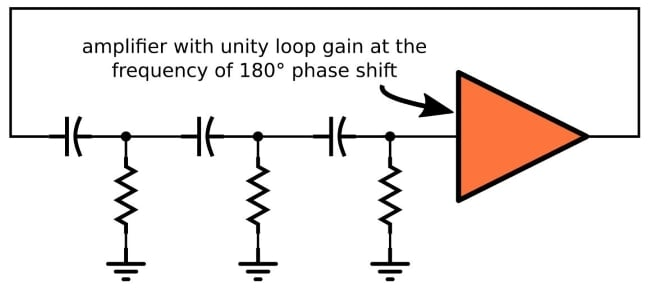This version uses three high-pass stages. For my LTspice simulations I created a modified implementation that is based on three low-pass stages: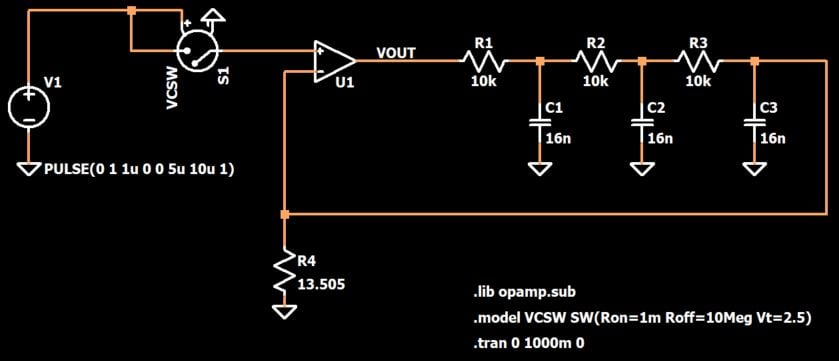The first article on this topic explained the general functionality of the circuit, but we still have some issues that need to be sorted out:

• What determines the frequency of oscillation?
• How can we analyze and understand the frequency response and stability characteristics?
• Stable oscillations can theoretically be obtained by setting R4 to the perfect value, but this would never work in real life. To turn this circuit into a practical oscillator, we need to incorporate a limiter.

### Frequency of Oscillation

As you can see in the circuit above, the phase-shift oscillator includes three RC low-pass filters, each of which has R = 10 kΩ and C = 16 nF. The cutoff frequency of each RC stage is 994 Hz (call it 1 kHz). It would be very convenient if this were also the frequency of oscillation, but this is not at all the case.

Let’s forget about the phase-shift oscillator for a moment and consider just the frequency response of this three-RC-stage low-pass filter: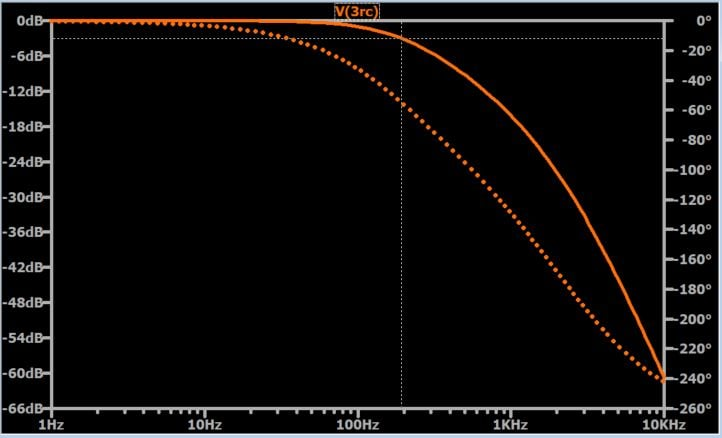As you know, another name for cutoff frequency is –3dB frequency. As shown by the cursor in the plot, the –3dB frequency of this filter is nowhere near 1 kHz. This occurs because the three stages interact with one another; a circuit consisting of three cascaded 1 kHz RC filters is not the same as one third-order 1 kHz filter. So you can’t choose your oscillation frequency simply by setting the cutoff frequency of the individual RC stages. And in case you’re wondering, the cutoff frequency of the overall three-stage circuit is also not the frequency of oscillation.

It’s important to remember that the cutoff frequency is not the critical parameter here. As discussed in the previous article, the critical parameter is phase shift. Oscillations occur at the frequency of 180° phase shift (f180), if the loop gain at this frequency is greater than unity.

The cursor in the following plot shows that the three-RC-stage filter has f180 ≈ 2.5 kHz.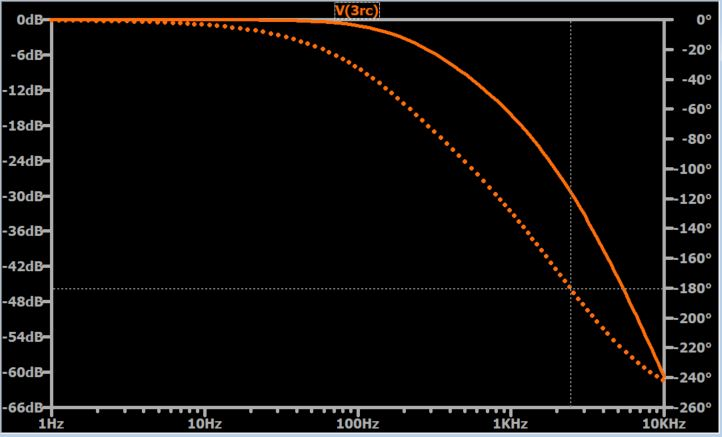So is 2.5 kHz the frequency of oscillation? Well, no. The trouble is that the three-RC-stage filter does not exist in isolation. It is connected to an op-amp and included in a negative-feedback path, and consequently the frequency of oscillation is determined by the interaction of this feedback network with the frequency-dependent behavior of the amplifier itself.

### Stability Analysis

In order to better understand this circuit’s oscillatory action, we need to perform some loop-gain-based stability analysis. If you have no idea what I’m talking about, you should read my article on stability and then the next on gain margin and phase margin. (Or even better, read the entire Negative Feedback series.)

The bottom line is that we’re going to simulate A (i.e., the open-loop gain of the op-amp) and β (i.e., the frequency response of the feedback network), then we will mathematically combine them into the loop gain Aβ. This is the circuit that we’ll use: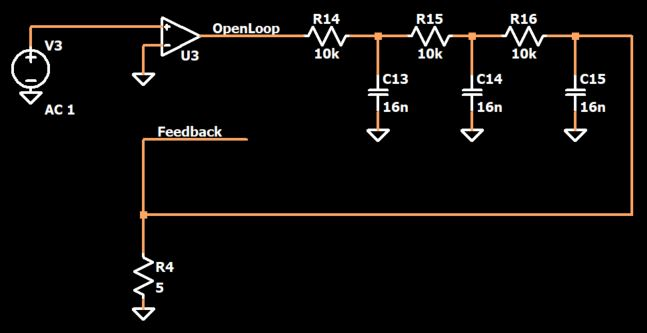The feedback response β is equal to the feedback voltage divided by the open-loop output voltage (when I say “feedback voltage”, I mean the voltage that would be fed back to the op-amp if the circuit were in a closed-loop configuration instead of an open-loop configuration); thus, “V(openloop)*(V(feedback)/V(openloop))” is equivalent to the loop gain Aβ.

Here is a plot for the above circuit.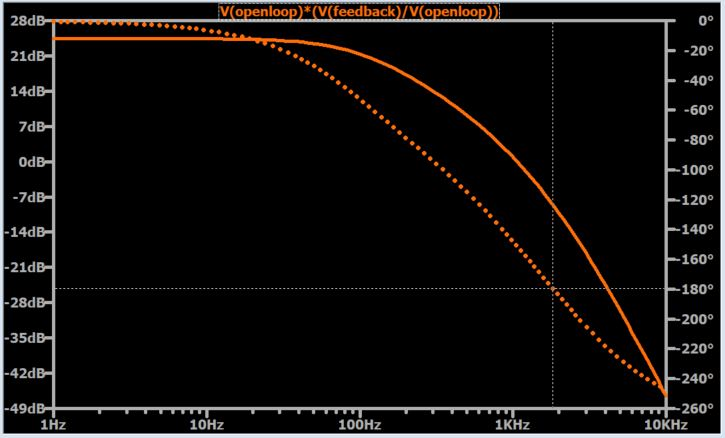The cursor shows that 180° phase shift occurs at ~1.8 kHz. Have we finally found the frequency of oscillation?

Yes! This is indeed the frequency at which the phase-shift oscillator will oscillate; however, the current circuit won’t oscillate because the magnitude of the loop gain is less than unity at f180.

From my time-domain experimentation, I discovered that oscillations transition from overdamped to underdamped when R4 is around 13.5 Ω. In other words, if R4 is less than 13.5 Ω, oscillations will gradually die away. If R4 is greater than 13.5 Ω, oscillations will increase in amplitude (until the circuit saturates). This means that an R4 value of 13.5 Ω should create a frequency response in which the loop-gain magnitude is very close to unity at f180, and this is exactly what we find in the following plot, which I obtained by re-simulating with R4 = 13.5 Ω.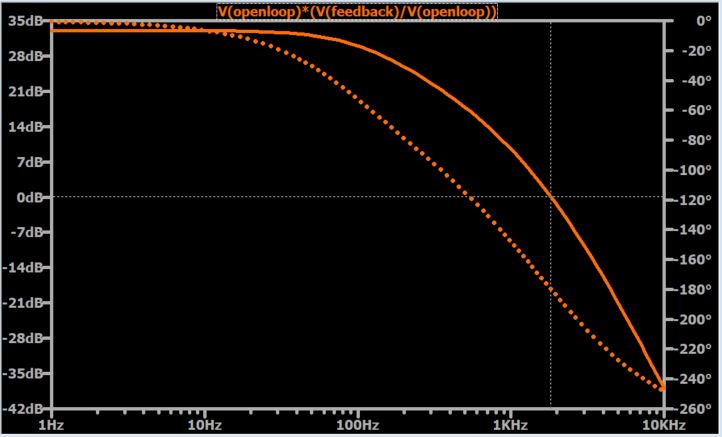The cursor marks the frequency at which the phase shift is 180°, and as you can see, the magnitude response at this frequency is very close to 0 dB.

We already covered the limiter circuit in a previous article, so all I really need to do at this point is show you the final circuit, i.e., a low-pass-filter-based phase-shift oscillator combined with a limiter. First, though, we should address one question: What value do we choose for R4?

We actually don’t want to use the ideal value because oscillations will be overdamped if environmental or operational variations cause the loop-gain magnitude to decrease slightly. We want a circuit that is slightly underdamped, so that oscillations start reliably and maintain their amplitude even if the loop gain changes somewhat; saturation will not occur because we have the limiter to keep everything under control. It’s important to note, however, that you don’t want the circuit to be excessively underdamped—the limiter will still prevent saturation, but the final waveform will have more distortion.

Here is the circuit: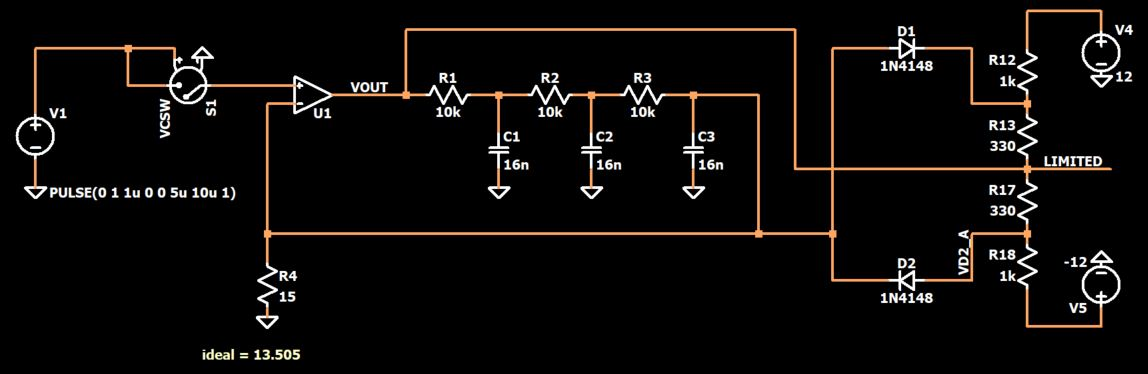##### Click to enlarge.

And here is the resulting sinusoid: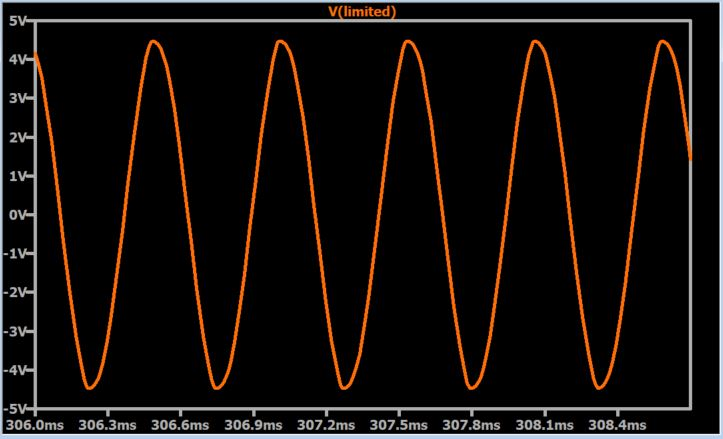The zoomed-out view confirms that the amplitude is stable: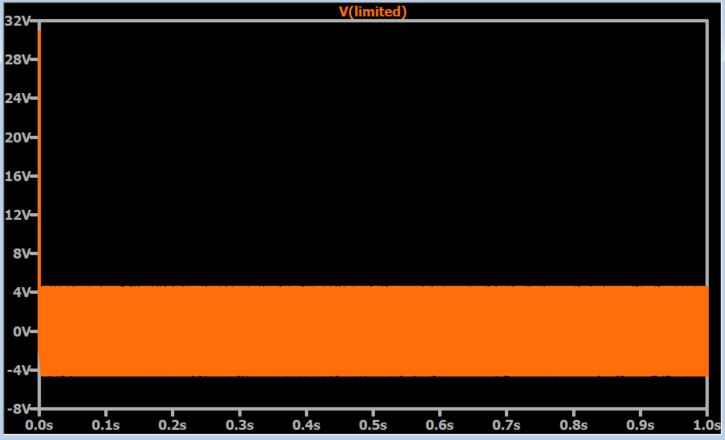I encourage you to download the LTspice file and experiment with different R4 values. If you look at the FFTs, you’ll see clearly that higher values of R4 lead to higher harmonic distortion in the sinusoid. You will also see that (sadly) the limiter circuit influences the frequency response, such that the oscillation frequency produced by the final circuit is even more difficult to predict.

### Conclusion

In this article, we took a detailed look at the frequency response and oscillation characteristics of a phase-shift oscillator, and we confirmed that the circuit can produce stable oscillations when combined with a limiter. If you want to continue analyzing and improving this circuit, you can download my LTspice schematic file by clicking on the orange button.

LPPhaseShiftOscillator_Part2.zip